• 算法分析与设计课件算法分析与设计课件算法分析与设计课件算法分析与设计课件
• ## 大学算法分析与设计复习总结

万次阅读 多人点赞 2013-06-08 11:49:20
大学算法分析与设计复习总结 为了拿大学的那悲剧的学分，好好弄懂以下所有知识点吧。把老师的复习的提纲，特意汇总了所有考点，方便童鞋们复习。不喜勿喷！！！ 这本书是《算法设计与分析》 王红梅 编著 一共...
大学算法分析与设计复习总结

为了拿大学的那悲剧的学分，好好弄懂以下所有知识点吧。把老师的复习的提纲，特意汇总了所有考点，方便童鞋们复习。不喜勿喷！！！
这本书是《算法设计与分析》 王红梅 编著
一共有以下12章，我们学了1、3、4、5、6、7、8、9
分别是“绪论、蛮力法、分治法、减治法、动态规划法、贪心法、回溯法、分治限界法

第1章 绪论
考点：

1、  算法的5个重要特性。（P3）
答：输入、输出、有穷性、确定性、可行性
2、  描述算法的四种方法分别是什么，有什么优缺点。（P4）
答：
1.      自然语言 优点：容易理解；缺点：容易出现二义性，并且算法都很冗长。
2.      流程图       优点：直观易懂；缺点：严密性不如程序语言，灵活性不如自然语言。
3.      程序设计语言 优点：用程序语言描述的算法能由计算机直接执行；缺点：抽象性差，是算法设计者拘泥于描述算法的具体细节，忽略了“好”算法和正确逻辑的重要性，此外，还要求算法设计者掌握程序设计语言及其编程技巧。
伪代码    优点：表达能力强，抽象性强，容易理解

3、  了解非递归算法的时间复杂性分析。(P13)
要点：对非递归算法时间复杂性的分析，关键是建立一个代表算法运行时间的求和表达式，然后用渐进符号表示这个求和表达式。
非递归算法分析的一般步骤是：
（1）      决定用哪个（或哪些）参数作为算法问题规模的度量。
（2）      找出算法的基本语句。
（3）      检查基本语句的执行次数是否只依赖问题规模。
（4）      建立基本语句执行次数的求和表达式。
（5）      用渐进符号表示这个求和表达式。

[例1.4]：求数组最小值算法
int ArrayMin(int a[ ], int n)
{
min=a;
for (i=1; i<n; i++)
if (a[i]<min) min=a[i];
return min;
}
问题规模：n
基本语句： a[i]<min
T(n)= n-1=O(n)

4、  掌握扩展递归技术和通用分治递推式的使用。（P15）
扩展递归技术：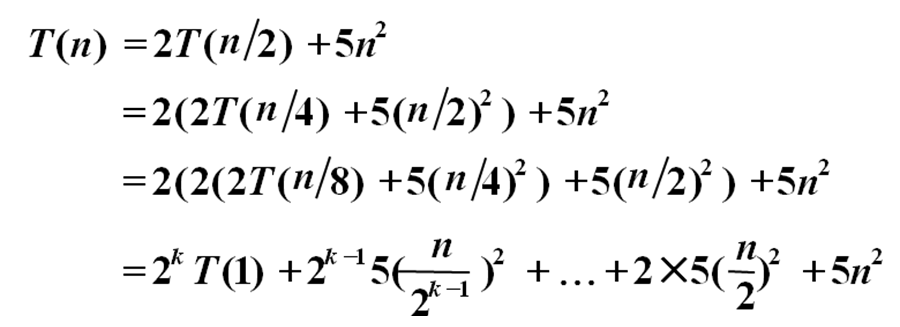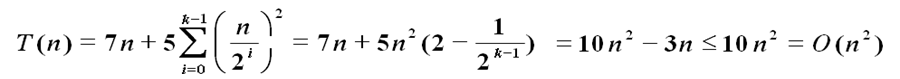通用分支递归式：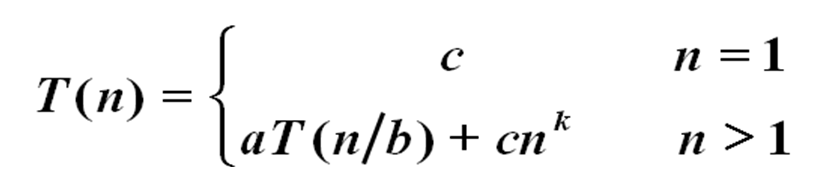5、  习题1-4，习题1-7
设计算法求数组中相差最小的两个元素（称为最接近数）的差。要求给出伪代码描述，并用一组例子进行跟踪验证，写出验证过程。
（1）伪代码
1.   令最小距离min等于数组头两个元素R和R的差的绝对值；
2.   从i=0循环至i<n-1，对于每个R[i]
2.1     分别求其与j=i+1至j<n的数的差的绝对值；
2.2     如果此值小于最小距离，则令新的最小距离为此值；
3.   输出最小距离。
（2）用实例进行跟踪验证
R={10,5,11,16,30,14},n=6;
Min=|10-5|=5;
i=0,j=1, |R[i]-R[j]|=|10-5|=5;
j=2,|R[i]-R[j]|=|10-11|=1<min;min=1;
j=3, |R[i]-R[j]|=|10-16|=6;
j=4, |R[i]-R[j]|=|10-30|=20;
j=5, |R[i]-R[j]|=|10-14|=4;
i=1,j=2, |R[i]-R[j]|=|5-11|=6;
j=3, |R[i]-R[j]|=|5-16|=11;
j=4, |R[i]-R[j]|=|5-30|=15;
j=5, |R[i]-R[j]|=|5-14|=9;
i=2,j=3, |R[i]-R[j]|=|11-16|=5;
j=4, |R[i]-R[j]|=|11-30|=19;
j=5, |R[i]-R[j]|=|11-14|=3;
i=3,j=4, |R[i]-R[j]|=|16-30|=14;
j=5, |R[i]-R[j]|=|16-14|=2;
i=4,j=5, |R[i]-R[j]|=|30-14|=16;
最后输出min=1

7、使用扩展递归技术求解下列递推关系式
（1）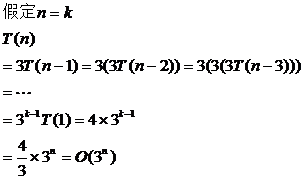（2）第3章 蛮力法

1、  掌握蛮力法的设计思想：
蛮力法依赖的基本技术——扫描技术，即采用一定的策略将待求解问题的所有元素依次处理一次，从而找出问题的解;
关键——依次处理所有元素。

2、  蛮力法的代表算法及其时间复杂度：
顺序查找，O(n)
串匹配（BF O(n*m) ，KMPO(n+m) ， BMO(n*m)）
选择排序，O(n2)
冒泡排序，O(n2)
生成排列对象（排列问题），O(n!)
生成子集（组合问题），O(2n)
0/1背包 属于组合问题。
任务分配，哈密顿回路，TSP问题 属于排列问题。
最近对问题 O(n2)，凸包问题 O(n3)
3、  掌握BF和KMP算法的原理，能够画出比较过程。P71习题3的4。要求给出一串字符串，能够求出对应的next数组，并能使用KMP算法进行比较匹配。
4、  掌握选择排序和冒泡排序算法描述和时间复杂性，要求能够写出伪代码。（P56-58）
选择排序
算法描述：选择排序开始的时候，扫描整个序列，找到整个序列的最小记录和序列中的第一记录交换，从而将最小记录放到它在有序区的最终位置上，然后再从第二个记录开始扫描序列，找到n-1个序列中的最小记录，再和第二个记录交换位置。一般地，第i趟排序从第i个记录开始扫描序列，在n-i+1个记录中找到关键码最小的记录，并和第i个记录交换作为有序序列的第i个记录。
时间复杂性：O(n2)
伪代码：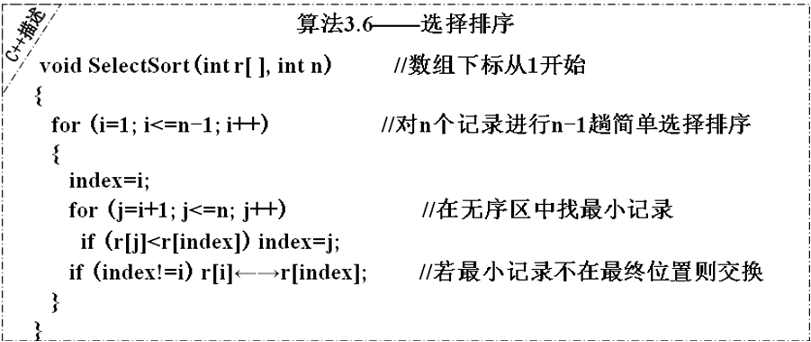冒泡排序
算法描述：冒泡排序开始的时候扫描整个序列，在扫描过程中两两比较相邻记录，如果反序则交换，最终，最大记录就能被“沉到”了序列的最后一个位置，第二趟扫描将第二大记录“沉到”了倒数第二个位置，重复上述操作，直到n-1趟扫描后，整个序列就排好序了。
冒泡排序，O(n2)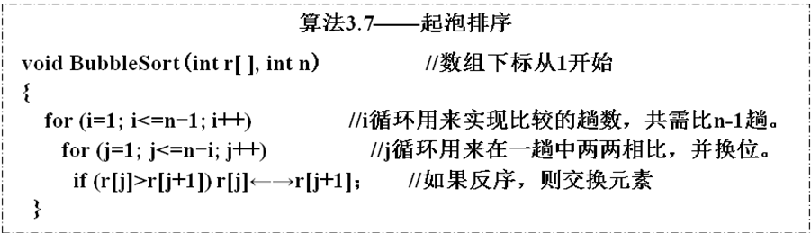5、  算法设计题：习题3-3，3-6，3-8，3-11，3-13
3-3 对于KMP算法中求next数组问题，设计一个蛮力算法，并分析其时间性能。

voidGetNext(char T[ ], int next[ ])
{
next=0;
next=1;
j=T,k=0;
for(;j>2;j--){
for(n=j-2;n>=1;n--){//n为要比较的前缀的最后一个字符的下标
m=j-n;//m为要比较的后缀的第一个字符的下标
for(i=1;i<=n;i++)
{
if(T[i]!=T[m+i-1])break;
}
if(i==n+1){next[j]=n+1;break;}
}
if(n==0)next[j]=1;
}
}
3-4 假设在文本“ababcabccabccacbab”中查找模式 “abccac”,求分别采用BF算法和KMP算法进行串匹配过程中的字符比较次数。由此可知，用BF算法一共要进行3+1+4+1+1+6+1+1+1+6=25次比较方能匹配出

KMP算法：next[]={,0,1,1,1,1,2};由此可知，用KMP算法一共要进行3+4+6+5=18次比较方能匹配出

参考代码如下：
排列最终存储在长度为n的阶乘，元素类型为指针的数组中，数组指向一个排列，具体的排列数据存储在数组中。

int fabs(int n)
{
int r=1;
for(inti=n;i>1;i--)
r=r*i;
return r;

}

//排列存储在数组中
void getArrangement(int**&s,int n)
{
int * p,*q;
int * *s1;
int i,j,k,l,m,o;
s=new int *;
s=newint;
s=1;
for(i=2;i<=n;i++)
{
j=0;
o=0;
m=fabs(i-1);
s1=newint *[fabs(i)];
while(o<m)
{
q=p=s[o];
for(k=i-1;k>=0;k--)
{
s1[j]=newint[i];
for(l=0;l<i;l++)
{
if(l==k){s1[j][l]=i;}
else{
s1[j][l]=*p;
p++;}
}
j++;
p=q;
}
o++;
delete[] q;
}
delete[]s;
s=s1;
}
}

3-8对于一个平面上n个点的集合S，设计蛮力算法求集合S的凸包的一个极点。
点集合中最左边或者最右边的点一定是凸包的一个极点，则求凸包的极点的问题转化为求点的x坐标最大或最小的点

int getPole(int x[],int y[],int n)
{
int r=0;
for(inti=0;i<n;i++)
{
if(x[i]>x[r])r=i;
}
return r;
} 

3-11 设计算法生成在n个元素中包含k个元素的所有组合对象。
两种思路：
1、  生成所有的组合，在组合中找元素个数为k个的组合。
伪代码：
1．初始化一个长度为n的比特串s=00…0并将对应的子集输出；
2．for(i=1; i<2n; i++)   //注意不能书写成i<=2n
2.1  s++；
2.2  判断s中1的个数，若为k，则将s对应的子集输出；

2、  使用k层嵌套循环生成元素个数为k个的组合。
设k=3；n个元素存储在数组a[]中；
伪代码：
for (i=1; i<n-2; i++)
for(j=i+1; i<n-1; i++)
for(k=j+1; i<n; i++)
输出a[i]a[j]a[k]构成的组合。

3-13美国有个连锁店叫7-11这个连锁店以前是每天7点开门，晚上11点关门
不过现在是全天24小时营业。有一天，有个人来到这个连锁店，买了4件商品
营业员拿起计算器敲了一下，说：总共是$7.11顾客开玩笑说：所以你们商店就叫7-11？营业员没有理她，说：当然不是，我是把它们的价格相乘之后得到的。 顾客说：相乘？你应该把他相加才对。营业员说，我弄错了。接着又算了一遍，结果让两个人吃惊的是：计算结果也是$7.11请问，这4件商品的价格是多少？
参考代码：

#include<iostream.h>
#include <stdio.h>
int main()
{
long i,j,k,m;

for (i=1; i <=711/4 ; i++)
{
for (j=i; j <=711/3 ; j++)
{
for (k=j; k <=711/2 ; k++)
{
m=711-i-j-k;
if (i*j*k*m==711*1000000)
{
cout<<i<<endl<<j<<endl<<k<<endl<<m<<endl;
}
}
}
}
return 0;
}输出结果为：价格分别是1.2   1.25   1.5     3.16

第4章  分治法

了解分治法的设计思想
设计思想：将要求解的原问题划分成k个较小规模的子问题，对这k个子问题分别求解。如果子问题的规模仍然不够小，则再将每个子问题划分为k个规模更小的子问题，如此分解下去，直到问题规模足够小，很容易求出其解为止，再将子问题的解合并为一个更大规模的问题的解，自底向上逐步求出原问题的解。
步骤：（1）划分（2）求解子问题（3）合并

分治法的代表算法及时间复杂度：
归并排序，快速排序，最大子段和，最近对问题，凸包问题，这五种问题的分治算法的时间复杂度为O(nlog2n)
棋盘覆盖，循环赛日程安排为O(4k)

掌握归并排序和快速排序算法的算法伪代码。（P78-83）
归并排序：算法中数组r中存储原始数据，r1在中间过程中存储排序后的数据，s指需排序数组的起始下标，t指需排序数组的结束下标。最终排序后的数据依然存储在r数组中。快速排序：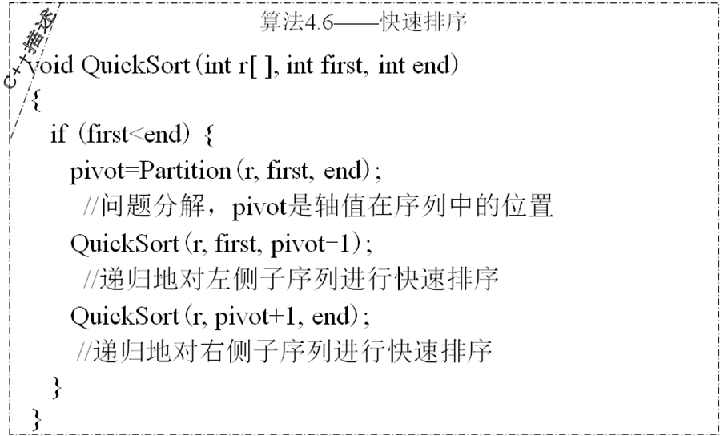掌握最大子段和问题的算法伪代码。（P83-85）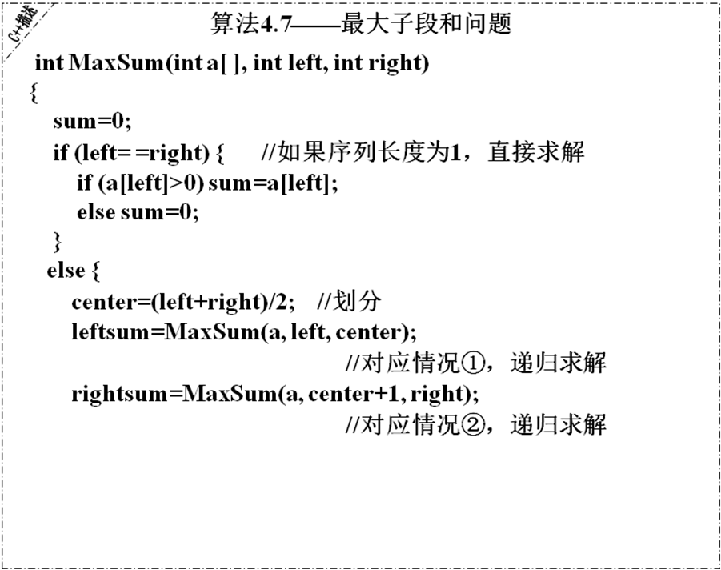对于待排序列（5, 3, 1, 9, 8, 2, 4, 7）,画出快速排序的递归运行轨迹。
按升序排列
初始序列：5,3,1,9,8,2,4,7
第一次划分：4,3,1,2,5,8,9,7
第二次划分：2,3,1,4,5,8,9,7
第三次划分：1,2,3,4,5,8,9,7
第四次划分：1,2,3,4,5,7,8,9
排序完成，红色字体为每次划分的轴值

在有序序列9（r1,r2,, rn）中，存在序号i ( 1<=i<=n),使得ri = i, 请设计一个分治算法找到这个元素，要求算法在最坏情况下的时间性能为O(log2n).
参考代码：

#include<iostream.h>
int findr(ints[],int begin,int end)
{
if(begin==end){
if(s[begin]==begin) return begin;
else return 0;
}else
{
int m=(begin+end)/2;
if(s[m]>m) return findr(s,begin,m-1);
else if (s[m]==m)return m;
else return findr(s,m+1,end);
}

}
void main()
{
int s[]={0,1,1,2,4,6,8};
cout<<findr(s,1,6)<<endl;
}

第5章 减治法

了解减治法的设计思想
设计思想：原问题的解只存在于其中一个较小规模的子问题中，所以，只需求解其中一个较小规模的子问题就可以得到原问题的解。

掌握使用减治法的代表问题及时间复杂度：
折半查找，二叉树查找，堆排序，选择问题，淘汰赛冠军问题，假币问题；
以上问题的时间复杂度，如果减治是每次减小为原来规模的1/2，则时间复杂度一般为O(log2n)

掌握折半查找的算法伪代码描述及具体例子的查找过程，会根据折半查找的过程创建判定树。（P98-100）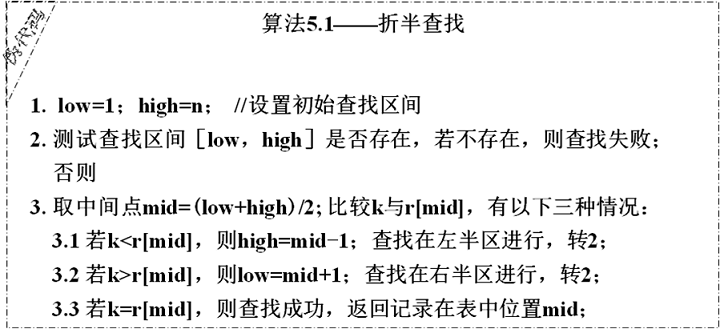会根据已知数据序列创建一个二叉查找树。（P100）掌握堆排序算法中的两种调整堆和新建堆的方法：筛选法和插入法（P101-105）
堆调整问题：将一个无序序列调整为堆
（1）筛选法调整堆
关键问题：完全二叉树中，根结点的左右子树均是堆，如何调整根结点，使整个完全二叉树成为一个堆？（2）插入法调整堆
关键问题是：在堆中插入一个结点，如何调整被插入结点，使整个完全二叉树仍然是一个堆？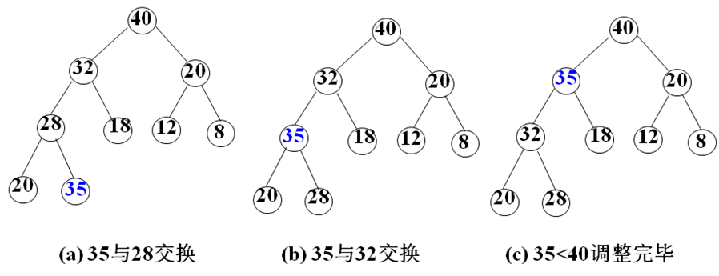掌握选择问题的算法的伪代码（P105-106）习题5-1，算法设计题
习题5-4，给出任意一组数据，能分别用筛选法和插入法写出创建堆的过程，并用两种方法进行堆排序。
对（47，5，26，28，10）进行筛选堆排序，使用大根堆，形成升序 ，列出每次筛选后的序列
形成大根堆的过程（先把数组直接表示成完全二叉树）：
47，5，26，28，10（叶子结点，不用筛选）
47，5，26，28，10 （叶子结点，不用筛选）
47，5，26，28，10 （叶子结点，不用筛选）
47，5，26，28，10
47，28，26，5，10 （5与两个孩子中的大者比较，5小，交换位置）
47，28，26，5，10 （47与两个孩子中的大者比较，47大，不用交换位置）
47,28, 26, 5 ,10 （大根堆）
10,28, 26, 5 , 47 （取出堆顶元素后的序列）
10,28, 26, 5 , 47 （筛选）
28, 10 , 26, 5 , 47
28, 10 , 26, 5 , 47 （大根堆）
5, 10 , 26, 28, 47 （取出堆顶元素后的序列）
5, 10 , 26, 28, 47 （筛选）
26, 10 , 5, 28, 47
26, 10 , 5, 28, 47 （大根堆）
5, 10 , 26, 28, 47 （取出堆顶元素后的序列）
5, 10 , 26, 28, 47 （筛选）
10, 5 , 26, 28, 47
10, 5 , 26, 28, 47 （大根堆）
5, 10 , 26, 28, 47 （取出堆顶元素后只剩一个值，结束算法）
对（47，5，26，28，10）进行插入法生成大根堆
47
47 5
47 5 26
47 28 26 5
47 28 26 5 10

第6章 动态规划法

了解动态规划法的设计思想
设计思想：将待求解问题分解成若干个相互重叠的子问题，每个子问题对应决策过程的一个阶段，将子问题的解求解一次并填入表中，当需要再次求解此子问题时，可以通过查表获得该子问题的解而不用再次求解。
步骤：
将原始问题分解为相互重叠的子问题，确定动态规划函数；
求解子问题，填表；
根据表，自底向上计算出原问题的解。

掌握可以用动态规划法解决的问题及时间复杂度：
TSP，多段图的最短路径问题，0/1背包，最长公共子序列问题，最优二叉查找树，近似串匹配问题；
多段图的最短路径问题： O(n+m)
0/1背包问题： O(n×C)

掌握多段图的最短路径问题的动态规划算法及具体实现（P121-123），习题6-2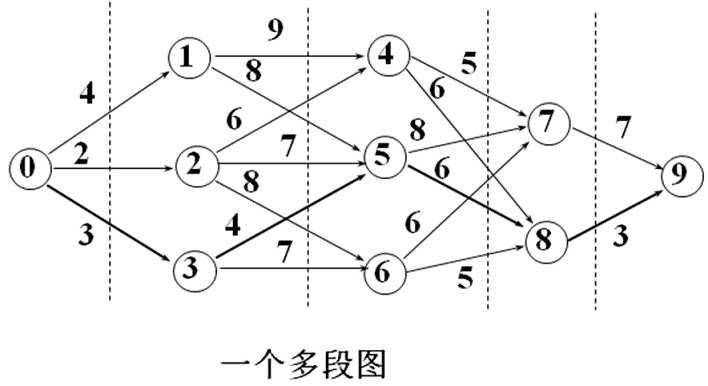动态规划函数为：
cost[i]中存储顶点i到终点的最短路径长度
cost[i]=min{C[i][j]+cost[j]} (i≤j≤n且顶点j是顶点i的邻接点)
path[i]=使C[i][j]+cost[j]最小的j
先构造cost数组和path数组掌握0/1背包问题的动态规划算法及具体实现（P123-126），习题6-3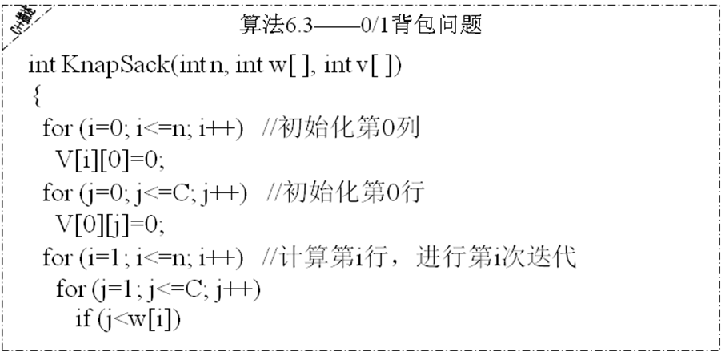例题：用动态规划法求如下0/1背包问题的最优解：有5个物品，其重量分别为（3，2，1，4，5），物品的价值分别为（25，20，15，40， 50），背包容量为6。写出求解过程。
0/1背包问题的动态规划函数为：V(i,j)表示把前i个物品放入容量为j的背包中的最大价值和。
填表过程：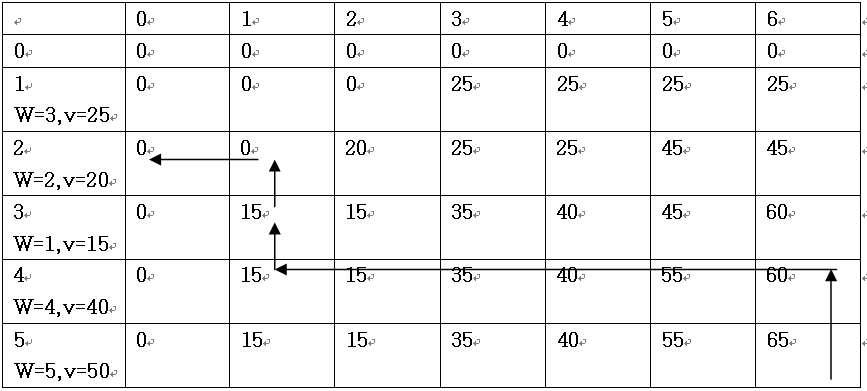放入背包中的物品的求解过程：则65表示把5个物品放入容量为6的背包中的最大价值和。
i=5,j=6;  v>v,x=1, j=6-w=1
i=4,j=1; v=v, x=0
i=3,j=1; v>v, x=1, j=1-w=0
i=2,j=0; v=v, x=0
i=1,j=0; v=v, x=0
结果是把第3个和第5个放入了背包
掌握最长公共子序列问题的动态规划法算法及具体实现（P126-128），习题6-4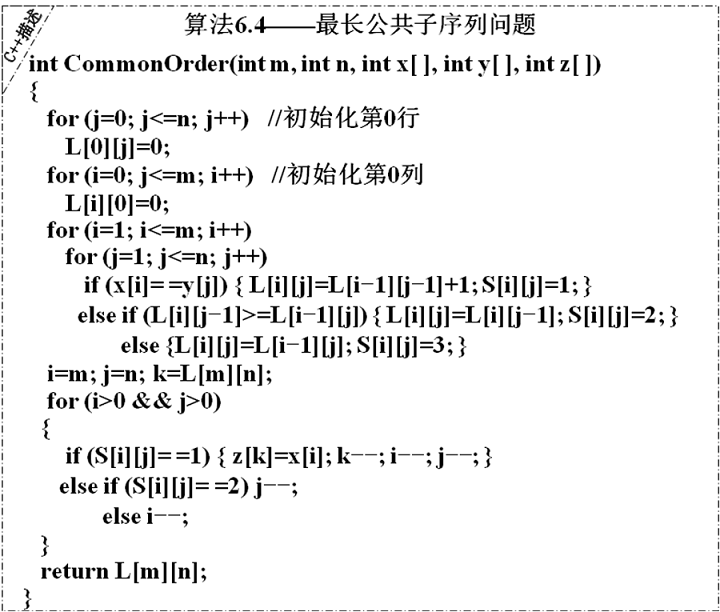求X=“xzyzzyx”和Y=“zxyyzxz”序列的最长公共子序列的动态规划函数为：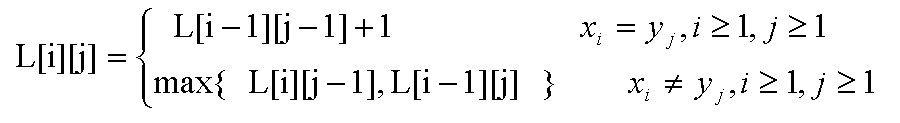L[i][j]表示X中前i个元素和Y中前j个元素构成的序列的最长公共子序列的长度。
为了确定具体的最长公共子序列，需要同时计算S[i][j]的值，S[i][j]表示在计算L[i][j]的过程中的搜索状态。子序列为斜箭头所标示的行或列：X,X,X ,X或Y, Y, Y , Y最长公共子序列的长度为4
即为：zyyx

第7章 贪心法
了解贪心法的设计思想

贪心法在解决问题的策略上目光短浅，只根据当前已有的信息就做出局部最优选择，而且一旦做出了选择，不管将来有什么结果，这个选择都不会改变。
贪心法的关键在于决定贪心策略。

掌握可以用贪心法解决的问题：
TSP问题中的两种解决方法：最近领点策略，最短链接策略
最小生成树问题的两种算法：最近顶点策略（Prim算法），最短边策略（Kruskal算法）
背包问题，活动安排问题，多机调度问题，哈夫曼编码。

掌握最小生成树的两种贪心算法：prim算法和kruskal算法（P145-148），给出具体的例子，能够用两种方法画出树的生成过程。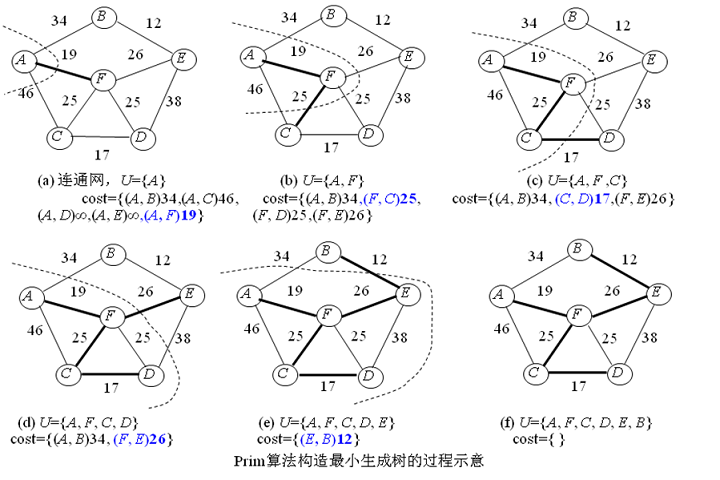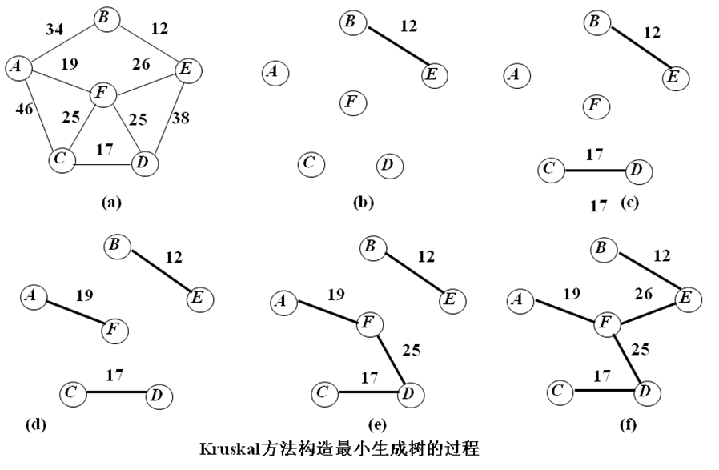掌握背包问题的贪心算法（P148-151），给出一个具体的例子，能够写出解决问题的过程。习题7-2
问题：求如下背包问题的最优解：有7个物品，价值P=(10,5,15,7,6,18,3),重量w=(2,3,5,7,1,4,1),背包容量W=15.
解决方法：
先对物品的单位重量价值按照降序排列

物品重量

物品价值

物品价值/物品重量

1

6

6

2

10

5

4

18

4.5

5

15

3

1

3

3

3

5

1.67

7

7

1

依次把物品放入容量为15的背包，直到背包被装满
1+2+4+5+1=13，前5个物品装入背包，还剩下容量为2，第6个物品只能装入2/3
所以总价值为：6+10+18+15+3+5*2/3=55.3333

给出字符集和对应的频率，能够画出对应的哈夫曼树，并对给定的字符串进行哈夫曼编码。（P155-157）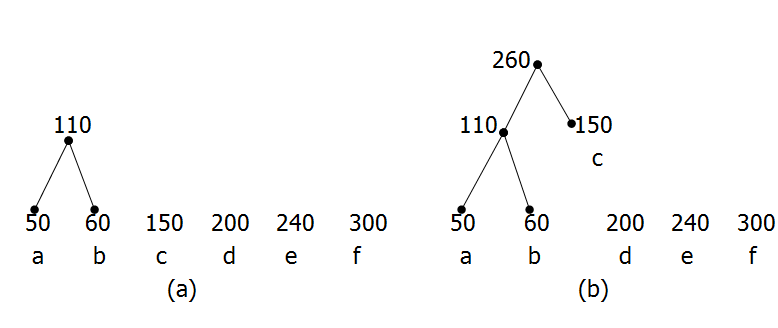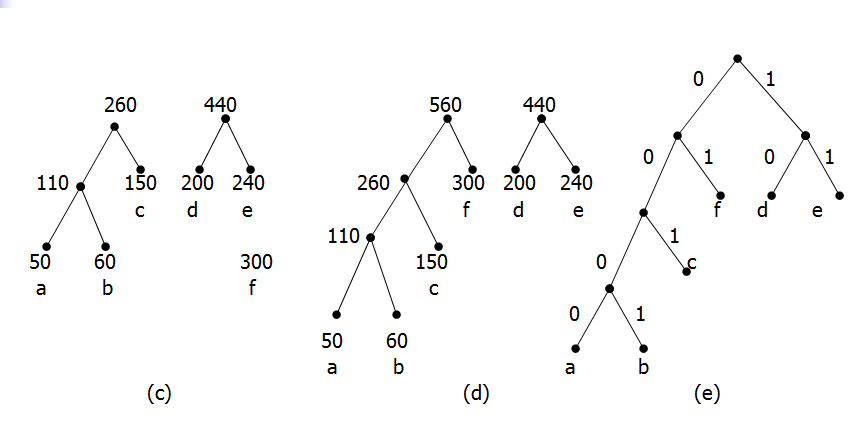第8章 回溯法
了解回溯法的设计思想
设计思想：从解空间树根结点出发，按照深度优先策略遍历解空间树，在搜索至树中任一结点时，先判断该结点对应的部分解是否满足约束条件，或者是否超出目标函数的界，也就是判断该结点是否包含问题的（最优）解，如果肯定不包含，则跳过对以该结点为根的子树的搜索，即所谓剪枝（Pruning）；否则，进入以该结点为根的子树，继续按照深度优先策略搜索。直到搜索到叶子结点，则得到问题的一个可能解。
步骤：
确定解向量和分量的取值范围，构造解空间树；
确定剪枝函数；
对解空间树按深度优先搜索，搜索过程中剪枝；
从所有的可能解中确定最优解。

了解可以用回溯法解决的问题：
属于组合问题和排列问题中求最优解的问题都可以用回溯法解决，例如：图着色问题，哈密顿回路问题，八皇后问题（4皇后问题），批处理作业调度问题。

掌握m颜色图着色判定问题的回溯法算法，并能画出解空间树的搜索过程（P168-170），习题8-4
对图8.14使用回溯法求解图问题，画出生成的搜索空间。解：图着色问题求解的是满足图着色要求的最小颜色数。对图8.14应该从1、2、3、4……种颜色依次尝试用回溯法判定是否满足M着色的要求。
经搜索，1种和2种颜色均不能满足图着色的要求，3种颜色可以满足图着色要求，搜索过程如下，所以图8.14的着色的最少颜色数应该为3
搜索空间为：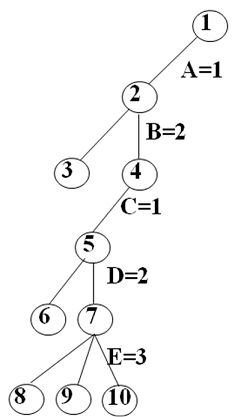掌握n皇后问题的回溯法算法，并能画出解空间树的搜索过程（P173-174）,
自己看书
掌握0/1背包问题的回溯算法，并能画出解空间树的搜索过程（P163-164），习题8-5
自己看书
习题8-6，算法设计题。
给定一个正整数集合X={x1,x2,…, xn}和一个正整数y，设计回溯算法，求集合X的一个子集Y，使得Y中元素之和等于y。
解：
用回溯法求解问题分析：
该问题为求子集问题。
解分量的和小于y为剪枝函数。
当搜索到结点，并且解分量的和等于y时，找到问题的解。

1．X={x1,x2,x3……xn }，sum=0，Y={ }为解向量，初始化为全0;
2．flag=false;
3．k=1;
4．while (k>=1)
4.1 当(Sk取1、0)循环执行下列操作
4.1.1yk=Sk中的下一个元素;
4.1.2将yk加入Y;
4.1.3sum=+（yk?xk:0）;
4.1.4if (sum==y) flag=true; 转步骤5;
4.1.4else if (sum<y) k=k+1; 转步骤4;
4.2 重置Sk，使得下一个元素排在第1位;
4.3 k=k-1;    //回溯
5．if flag 输出解Y;
else 输出“无解”;
参考代码：

#include <iostream.h>
const int N=5;
int f(int x[],int  y[],int n)
{
//初始化y，y为所求的集合
for(inti=0;i<N;i++)
y[i]=2;
int k=0;
int sum=0;
while(k>=0)
{
y[k]=y[k]-1;
if((y[k]==1||y[k]==0)&&k<N){
sum=sum+(y[k]?x[k]:0);
if(sum==n){break;}//找到解
else{
if(sum<n){k++;}//搜索下一个
else{
sum=sum-(y[k]?x[k]:0);
}
}
}
else{//回溯
//  sum=sum-(y[k]?x[k]:0);
y[k]=2;
k--;
sum=sum-(y[k]?x[k]:0);
}
}
return k;
}

void main()
{
int x[N]={2,1,3,4,2};
int y[N]; //解向量
int n=12; //题目要求等于的和
int k=f(x,y,n);//k表示搜索到第几个元素
cout<<k<<endl;
for(int i=0;i<N;i++)
cout<<(y[i]==1?x[i]:0)<<endl;

}第9章 分治限界法
了解分支限界法的设计思想
设计思想：
1）首先确定一个合理的限界函数，并根据限界函数确定目标函数的界[down, up] ，并确定限界函数；
2）然后按照广度优先策略遍历问题的解空间树，在分支结点上，依次搜索该结点的所有孩子结点，分别估算这些孩子结点的限界函数的可能取值；
3）如果某孩子结点的限界函数可能取得的值超出目标函数的界，则将其丢弃；否则，将其加入待处理结点表（以下简称表PT）中；
4）依次从表PT中选取使限界函数的值是极值的结点成为当前扩展结点；
5）重复上述过程，直到找到搜索到叶子结点，如果叶子结点的限界函数的值是极值，则就是问题的最优解，否则，找到其他极值结点重复扩展搜索。
步骤：
确定解空间树
确定限界函数
按广度优先搜索解空间树，计算限界函数的值，填入PT表
从PT表中寻找极值，继续扩展结点，直到找到限界函数值为极值的叶子结点。

了解可以使用分支限界法解决的问题：
TSP问题，多段图的最短路径问题，任务分配问题，批处理作业调度问题，0/1背包问题。
掌握任务分配问题的分支限界法（P195-197），习题9-5
掌握0/1背包问题的分支限界法（P184-185），习题9-6
掌握批处理作业问题的分支限界法（P198-200），习题9-7


展开全文编程语言
• 算法分析与设计
算法分析与设计-----递推算法
递推法是一种重要的数学方法，在数学的各个领域中都有广泛的运用，也是计算机用于数值计算的一个重要算法。
这种算法特点是：
一个问题的求解需一系列的计算，
在已知条件和所求问题之间总存在着某种相互联系的关系，
如果可以找到前后过程之间的数量关系（即递推式），那么，从问题出发逐步推到已知条件，此种方法叫逆推。
无论顺推还是逆推，其关键是要找到递推式。
斐波那契数列问题
Fibonacci 数列：0，1，1，2，3，5，8，13，21，34，……
f0 = 0
f1 = 1
fn = fn-1 + fn-2	( n >= 2 )
分析：可以用迭代方法求解，为了得到当前项，要使用前两项，所以用两个变量迭代。
代码：
#include <iostream>
using namespace std ;
int main(){
int n, i, a0, a1 ;
cout << "n = " ;
cin >> n ;
a0 = 0 ;  a1 = 1 ;
cout << a0 << "  "<< a1 << "  ";
for ( i = 2; i <= n/2 ; i ++ ){
a0 = a0 + a1 ;
a1 = a1 + a0 ;
cout << a0 << "  "<< a1 << "  ";
if ( i % 5 == 0 )  cout << endl ;
}
if ( n > (i-1)*2 )  cout << a0+a1 << endl ;
return 0;
}


汉诺塔移动次数问题
规则：一次只能移动一个盘子，小的盘子必须放在大的盘子上。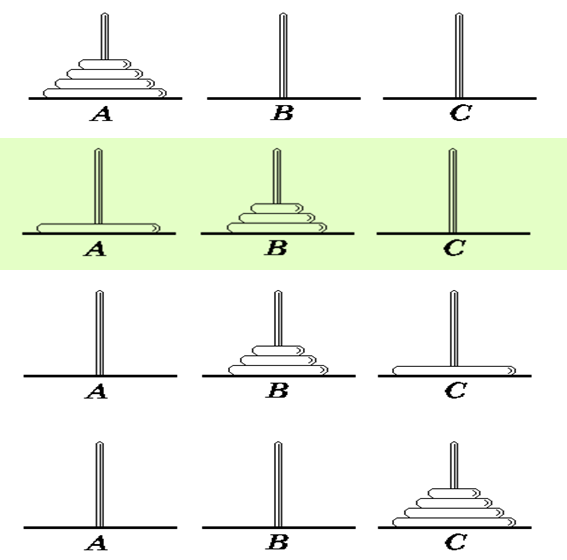递推关系分析
f(n)=2*f(n-1)+1
边界条件：f(1)=1
#include <iostream>
using namespace std;
int main(){
int f={0,1};
int n;
cin>>n;
for(int i=2;i<=n;i++)
f[i]=2*f[i-1]+1;
cout<<f[n]<<endl;
return 0;
}

猴子吃桃问题
问题描述：
猴子第一天采摘了一些桃子，第二天吃了第一天的一半多一个，第三天吃了第二天的一半多一个…直到第十天就剩下一个。问：猴子第一天摘了多少桃子？
分析：递推关系:
f(n)=f(n-1)/2-1
f(n-1)=(f(n)+1)*2
边界条件：f(10)=1
代码
#include <iostream>
using namespace std;
int main(){
int f;
f=1;
for(int i=9;i>=1;i--)
f[i]=2*(f[i+1]+1);
cout<<f<<endl;
return 0;
}



展开全文• 算法分析与设计内容包含：递归与分治，动态规划，贪心算法
• 算法分析与设计 递归 递归的基本概念 · 递归是指在函数的定义中调用函数自身，一个经典的例子就是用递归函数求阶乘 int factorial(int n){ if(n == 0){ return 1; }else{ return n * factorial(n-1); } } ​ ...算法分析与设计 递归
递归的基本概念
·	递归是指在函数的定义中调用函数自身，一个经典的例子就是用递归函数求阶乘
int factorial(int n){
if(n == 0){
return 1;
}else{
return n * factorial(n-1);
}
}

​	从上例可以看出递归的基本思想就是把规模大的问题拆解为规模小的相同的子问题来解决，且在这个不断拆解为更小问题的过程中有一个临界点，即问题拆解的终止条件。当达到临界点时，从被拆解的最小问题的解开始返回答案，累积得到原问题的解。
​	所以在递归函数实现时，包含了两部分，一个是递归主体，另一个是终止条件。因为大问题和小问题是一样的问题，因此大问题的解决方法和小问题的解决方法也是同一个方法这就是递归主体。这种函数调用它自身的情况，必须有明确的终止条件，否则就会导致无限递归。
​	总的来说，递归问题的求解关键在于找出问题转化的递推公式和终止条件，递推公式来自将大问题拆解为小问题的规律，终止条件就是问题的平凡解，即问题的最简单情况。
​	递归的作用：

替代多重循环
解决本来就是用递归形式定义的问题
将问题分解为规模更小的子问题进行求解

汉诺塔问题
​	有一个梵塔，塔内有三个座 A 、 B 、 C，A 座上有 64 个盘子，盘子大小不等，大的在下，小的在上。 现在想把这 64 个盘子从 A 座移到 B 座，但每次只能允许移动一个盘子，并且在移动过程中， 3 个座上的盘子始终保持大盘在下，小盘在上 。 在移动过程中可以利用 C 座，要求移动的步骤。分析问题：

初始情况：将圆盘按大小顺序堆放在 A 上，最大的在底部
移动规则：允许圆盘一次从一个木桩移动到另一个，大盘不能放在小盘的上面
最终目标：以最少的移动次数将所有圆盘从 A 转移到 B
终止条件：A 只有一个盘子，直接将它从 A 移动到 B
递推规律：先将 n-1 个盘子从 A 移动到中转 C，再将最大的一个盘子移动到 B，然后将 n-1 个盘子从 C 移动到 B

#include<iostream>
using namespace std;

void hanoi(int n, char src, char mid, char dest){
if(n == 1){
cout << src << "->" << dest << endl;
return; // 终止条件
}
hanoi(n-1, src, dest, mid); // 将 n-1 个盘子从 src 移到 mid 柱
cout << src << "->" << dest << endl; // 将 src 柱的最大盘移到 dest 柱
hanoi(n-1, mid, src, dest); // 将 n-1 个盘子从 mid 移到 dest 柱
}

int main(){
int n = 3;
hanoi(n,'A','C','B');
return 0;
}

八皇后问题
​	在8×8格的国际象棋上摆放八个皇后，使其不能互相攻击，即任意两个皇后都不能处于同一行、同一列或同一斜线上，问一共有多少种摆法。分析问题：

初始情况：8X8的棋盘上，待放入八个皇后棋子
放入规则：任意两个皇后棋子都不能处于同一行、同一列或同一斜线上
最终目标：将所有8个皇后棋子都放到棋盘上
终止条件：棋盘只有一个位置可以放入棋子
递推规律：先放第一个皇后，然后将第二个皇后放入到剩下7X7的棋盘上不会被攻击的位置，然后将第三个皇后放入到剩下6X6的棋盘上不会被攻击的位置，直到放完所有棋子

#include<iostream>
#include<vector>
using namespace std;

int n = 8;
vector<int> queenPos(100);

void nQueen(int k){
// 第 n 个皇后放入完毕，输出一种摆放方案
if(k == n){
for(int i=0; i<n; ++i){
cout << queenPos[i]+1 << ' ';
}
cout << endl;
return;
}

// 放入第 k 个皇后
for(int i=0; i<n; ++i){
// 判断放入第 k 个皇后的位置和之前 k-1 个皇后的位置是否冲突
int j = 0;
for(j; j<k; ++j){
// 任意两个皇后棋子都不能处于同一行、同一列或同一斜线上
if(i == queenPos[j] || abs(k-j) == abs(queenPos[j]-i)){
break;
}
}
if(j == k){
queenPos[k] = i;
nQueen(k+1);
}
}
}

int main(){
nQueen(0);
return 0;
}

计算逆波兰表达式
​	逆波兰表达式是一种把运算符前置的算术表达式，例如普通的表达式 2 + 3 的逆波兰表示法为 2 3 + 。逆波兰表达式的优点是运算符之间不必有优先级关系，也不必用括号改变运算次序，例如 (2 + 3) * 4 的逆波兰表示法为 2 3 4 + * 。设计算法计算逆波兰表达式的值，其中运算符包括 +, -, *, /
分析问题：

终止条件：逆波兰表达式内容计算完成
递推规律：一个数是一个逆波兰表达式，值为该数；*逆波兰表达式 逆波兰表达式 运算符 *  这种递归定义也是逆波兰表达式，值为两个逆波兰表达式的值运算的结果。

#include<iostream>
#include<vector>
#include<string>
#include<cstring>
using namespace std;

double exp(vector<string>& notaion){
string term = notaion.back();
notaion.pop_back();
const char* c = term.data();
switch (c)
{
case '+':
return exp(notaion) + exp(notaion);
case '-':
return exp(notaion) - exp(notaion);
case '*':
return exp(notaion) * exp(notaion);
case '/':
return exp(notaion) / exp(notaion);
default:
return atof(c);
}
}

// 字符串分割函数
vector<string> split(const string& str, const string& delim){
vector<string> res;
if(""==str) return res;
// 先将 string 转化为 char*
char* strs = new char[str.length()+1];
strcpy(strs,str.c_str());
const char* dl = delim.c_str();
char *tokenPtr = strtok(strs,dl); // 使用 strtok() 函数分割字符串
while(tokenPtr){
string s = tokenPtr;
res.push_back(s);
tokenPtr = strtok(NULL,dl);
}

return res;
}

int main(){
string strNon = "35.0 24.0 + 12.0 11.0 + *";
vector<string> notation;
notation = split(strNon," ");
cout<< strNon << " = " << exp(notation) << endl;

return 0;
}

计算四则运算表达式
​	计算数学中最常见的四则运算表达式，表达式仅由整数和加减乘除构成，即只包含int, +, -, *, /,(, )这些内容，计算四则运算表达式的值。假设运算符结果都是整数，除法结果也是整数。
分析问题：

终止条件：四则运算表达式所有内容计算完成
递推规律：四则运算表达式的是递归定义的，表达式由参与加减的项构成，项由参与乘除的因子构成，因子可能由带括号的子表达式构成。对于表达式的递归分析如下图所示：#include<iostream>
#include<deque>
#include<string>
#include<cstring>
using namespace std;

int factor_value(deque<string>& exp);
int term_value(deque<string>& exp);

// 求一个表达式的值
int expression_value(deque<string>& exp){
int result = term_value(exp);
while (true)
{
string op = exp.front(); // 查看下一个字符
if(op =="+" || op == "-"){
exp.pop_front();
int value = term_value(exp);
if(op == "+"){
result += value;
}else{
result -= value;
}
}else{
break;
}
}

return result;
}

// 求一个项的值
int term_value(deque<string>& exp){
int result = factor_value(exp);
while(true){
string op = exp.front(); // 查看下一个字符
if(op =="*" || op == "/"){
exp.pop_front();
int value = factor_value(exp);
if(op == "*"){
result *= value;
}else{
result /= value;
}
}else{
break;
}
}
return result;
}

// 求一个因子的值
int factor_value(deque<string>& exp){
int result = 0;
string c = exp.front(); // 查看下一个字符
if(c == "("){
exp.pop_front();
result = expression_value(exp);
exp.pop_front();
}else{
const char* vc = c.data();
result += atoi(vc);
exp.pop_front();
}
return result;
}

// 字符串分割函数
deque<string> split(const string& str, const string& delim){
deque<string> res;
if(""==str) return res;
// 先将 string 转化为 char*
char* strs = new char[str.length()+1];
strcpy(strs,str.c_str());
const char* dl = delim.c_str();
char *tokenPtr = strtok(strs,dl); // 使用 strtok() 函数分割字符串
while(tokenPtr){
string s = tokenPtr;
res.push_back(s);
tokenPtr = strtok(NULL,dl);
}

return res;
}

int main(){
string expStr = "( 2 + 3 ) * ( 5 + 7 ) + 9 / 3";
deque<string> expDe = split(expStr," ");
cout << expression_value(expDe) << endl;
return 0;
}

爬楼梯问题
​	爬楼梯是指一个人爬楼梯，他可以每次可以走 1 级或者 2 级，输入楼梯的级数，求不同的走法总数。分析问题：
​	可以将原问题转化为子问题，再有子问题的解得出原问题的解，也可以使用递归的思想。

终止条件：本题可以有多种边界条件，n<0时没有台阶可走，n=0时需要返回上一步移动的结果，n=1时只剩下一种移动方式，n=2时有11和2两种移动方式。(1) n < 0, return 0; n = 0, return 1; (2) n = 0, return 1; n = 1, return 1; (3) n = 1, return 1; n = 2, return 2;
递推规律：(n 阶楼梯的走法) = (走一级台阶之后，n-1 级台阶的走法) + (走两级台阶之后，n-2 级台阶的走法)，形式化表达 f(n) = f(n-1) + f(n-2)

#include<iostream>

using namespace std;

int stairs(int n){
if(n == 0 || n == 1){
return 1;
}
return stairs(n-1) + stairs(n-2);
}

int main(){
int n = 8;
cout << stairs(n) << endl;
}

放苹果问题
​	把 M 个同样的苹果放在 N 个同样的盘子里，允许有的盘子空着不放，计算共有多少种不同的分法？ 5 1 1 和 1 5 1 视为同一种分法。分析问题：
​	使用递归的方法将原问题转化为子问题，在转化位子问题时注意考虑将问题分类

终止条件：所有的苹果放完了 M=0 则形成了一种有效的分法 return 1；所有的盘子用完了 N=0，但是苹果还没放完则是一种无效的分法 return 0
递推规律：设 i 个苹果放在 k 个盘子里放法总数是 f(i,k)，则可分为两种情况：(1) 盘子数大于苹果数，由于盘子不区分，所以这种情况直接可以视为盘子树和苹果数相等的情况，f(i,k) = f(i,i) (2) 盘子数小于等于苹果数，则(总的分法) = (有空盘子分法) + (无空盘子分法)，f(i,k) = f(i,k-1) + f(i-k,k); 有空盘子分法最少有一个空盘子，无空盘子分法每个盘子至少有一个苹果。

#include<iostream>
using namespace std;

int putApple(int ap, int pl){
if(ap < pl){
return putApple(ap,ap);
}else{
if(ap == 0){
return 1;
}
if(pl <= 0){
return 0;
}
}

return putApple(ap, pl-1) + putApple(ap-pl,pl);
}

int main(){
int ap = 7, pl = 3;
cout<<putApple(ap,pl)<<endl;
return 0;
}

算24问题
​	给出 4 个小于 10 个正整数，你可以使用加减乘除 4 种运算以及括号把这 4 个数连接起来得到一个表达式。问：是否存在一种方式使得得到的表达式的结果等于 24 。比如，对于 5 5 5 1 ，有 5 * (5 - 1 / 5) = 24 ，因此可以得到 24 ；而对于 1 1 4 2 ，怎么都不能得到 24 。
分析问题：

输入：输入数据包括一组测试数据，有 4 个小于 10 个正整数。
输出：对于一组测试数据，如果可以得到 24 ，输出 YES”，否则，输出“ NO” 。
终止条件：只用一个数算24，是24则返回true，不是则返回false
递推规律：n 个数算 24 ，必有两个数要先算。这两个数算的结果，和剩余 n-2 个数，就构成了 n-1 个数求 24 的子问题。
注意点：对于第一步算两个数，要枚举先算的两个数，以及这两个数的运算方式。除法运算中除数不能为0，而且形成浮点数相等比较不能直接用==运算符

#include<iostream>
#include<vector>
#include<cmath>
using namespace std;
vector<double> termArr(5);
// 浮点数相等比较
bool isEqual(double param1, double param2){
return fabs(param1-param2) < 0;
}

bool count24(vector<double>& term, int n){
// 边界条件，一个数算 24
if(n == 1){
if(isEqual(termArr,24)){
return true;
}else{
return false;
}
}

// 算两个数
vector<double> tempArr(5);
for(int i=0; i<n-1; ++i){
for(int j=i+1; j<n; ++j){
// 将剩下的数放到 tempArr中
int m = 0;
for(int k = 0; k < n-2; ++k){
if(k !=i && k != j){
tempArr[m++] = termArr[k];
}
}
// 遍历所有两个数的运算组合, 将运算结果添加到 tempArr 中
tempArr[m] = termArr[i] + termArr[j];
if(count24(tempArr,m+1)){
return true;
}
tempArr[m] = termArr[i] - termArr[j];
if(count24(tempArr,m+1)){
return true;
}
tempArr[m] = termArr[j] - termArr[i];
if(count24(tempArr,m+1)){
return true;
}
tempArr[m] = termArr[j] * termArr[i];
if(count24(tempArr,m+1)){
return true;
}
if(!isEqual(tempArr[j],0)){
tempArr[m] = termArr[i] / termArr[j];
if(count24(tempArr,m+1)){
return true;
}
}
if(!isEqual(tempArr[i],0)){
tempArr[m] = termArr[j] / termArr[i];
if(count24(tempArr,m+1)){
return true;
}
}
}
}
}

int main(){
termArr = {5,5,5,1};
if(count24(termArr,4)){
cout << "Yes" << endl;
}else{
cout << "No" << endl;
}
return 0;
}

Reference
逆波兰表达式计算

`
展开全文• 算法分析与设计电路布线问题算法分析与设计电路布线问题算法分析与设计电路布线问题算法分析与设计电路布线问题算法分析与设计电路布线问题算法分析与设计电路布线问题电路布线
• 计算机算法分析与设计复习1(基本算法框架）. 计算机算法分析与设计复习2(一题多解，全面复习）. 计算机算法分析与设计复习3(排序算法的复习）. 计算机算法分析与设计复习4（各类题型，逐一击破）. ...
• huffman树，算法分析与设计huffman树，算法分析与设计huffman树，算法分析与设计huffman树，算法分析与设计huffman树，算法分析与设计
• 算法分算法分算法分析与设计综合实例析与设计综合实例析与设计算法算法分析与设计综合实例分析与设计综合实例综合实例算法算法分析与设计综合实例分析与设计综合实例算法分析算法分算法分析与设计综合实例析与设计...
• 计算机算法分析与设计第四版配套课件，均为PPT。。。。
• 算法分析与设计，屈婉玲等编，课下习题答案 算法分析与设计，屈婉玲等编，课下习题答案 算法分析与设计，屈婉玲等编，课下习题答案
• Python算法分析与设计：随机算法 一、实验目的 1、了解两种典型的随机算法：蒙特卡洛和拉斯维加斯算法，以及它们之间的异同 2、熟练掌握利用随机算法求解典型的计算问题，如矩阵乘积结果验证、快速排序、选择第K小的...python 随机算法
• 计算几何——算法分析与设计第三版 完整 周培德 计算几何——算法分析与设计第三版 完整 周培德
• 算法分析与设计 二分查找 二分查找的基本概念 ​ 二分查找是一种在有序数组中查找某一特定元素的查找算法。这种查找方法将查找的时间复杂度从原本的线性时间提升到了对数时间范围，大大缩短了搜索时间。 ​ 二分查找...数据结构 二分查找 二分法
• 算法分析与设计的课件，包括动态规划、贪心算法，回朔限界法
• 算法分析与设计总结 回顾这八周以来关于这课程的学习情况，我体会最深的是：不论是从深度还是从广度上，现在所习的算法比以前学习的算法难度增加了很多。但是费老师极富经验的教学和课件，为我的学习提供了很大的...
• 算法分析与设计实验报告——二分搜索算法的实现 目录：算法分析与设计实验报告——二分搜索算法的实现一、 实验目的二、实验要求三、 实验原理四、 实验过程(步骤)五、 运行结果六、实验分析与讨论七、实验特色与...c++
• Python算法分析与设计：最大流算法 一、实验目的 1、掌握最大流问题的定义，了解流量、容量以及他们之间的关系。 2、掌握通过增广路径求最大流问题的Forder-Fulkerson和Edmond-Karp算法，理解这两个算法之间的异同 3...python
• 算法分析与设计课程总结 经过8周的学习，我对算法有了更深入的理解。代码水平也有了显著的提高。 我们学习的算法有：递归与分治策略，贪心算法，回溯算法，分支限界算法和动态规划算法。一、递归与分治策略 （一...
• 算法分析与设计 哈夫曼编码 问题描述： 代码（码字）：Q{001，00，010，01}表示字符a，b，c，d 同一序列：0100001 产生两种译码（产生歧义）：01 00 001；010 00 01 二元前缀码：任何字符的代码不能作为其他字符代码...
• Python算法分析与设计实验：贪心算法 一、实验目的 1、了解贪心算法求解优化问题的过程 2、熟练掌握利用贪心算法求解典型的计算问题，如硬币找零、间隔任务规划等问题。了解利用替换法证明贪心策略是否能获得全局最...python 贪心算法...